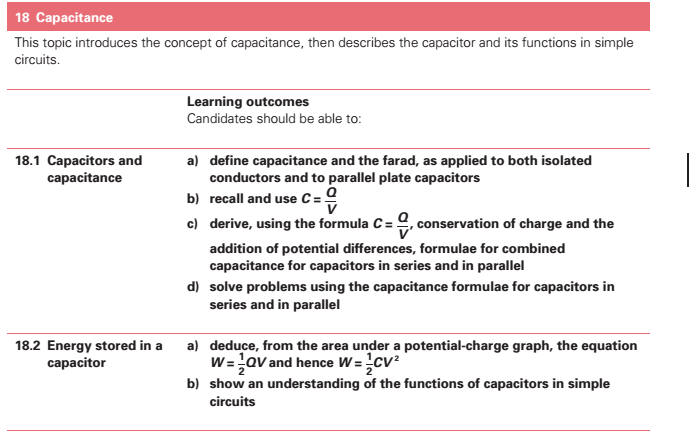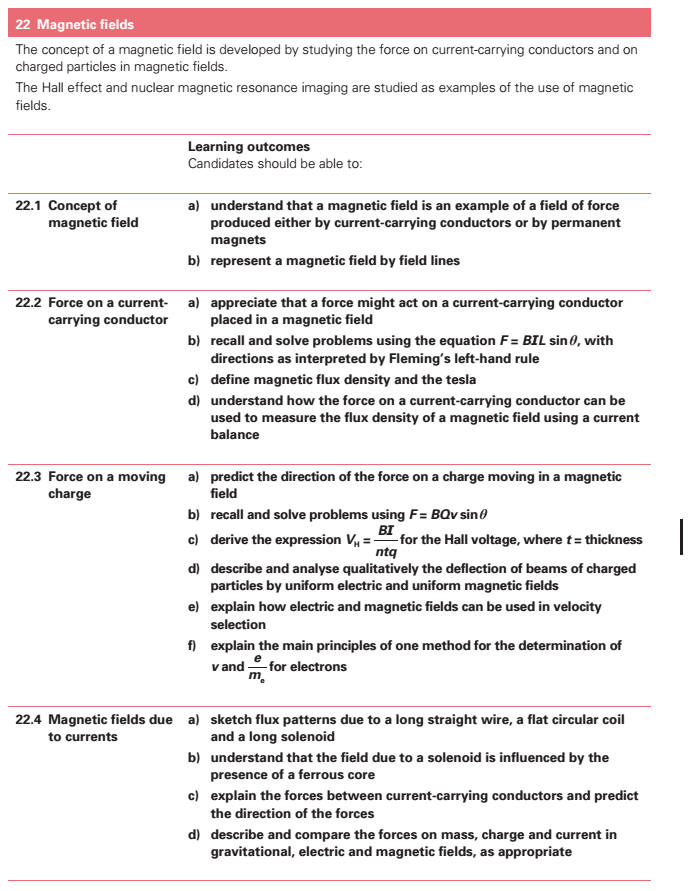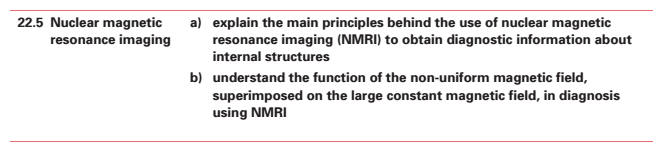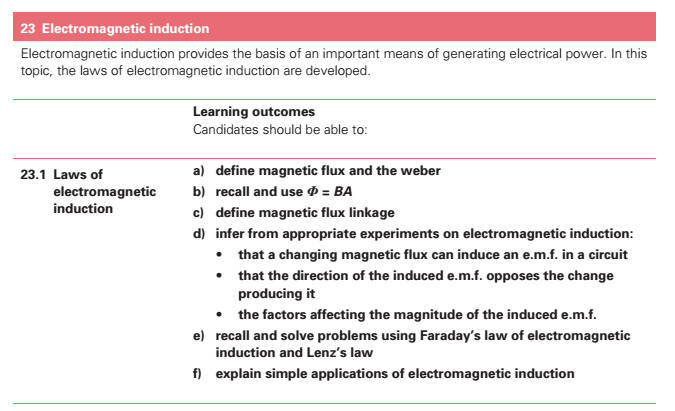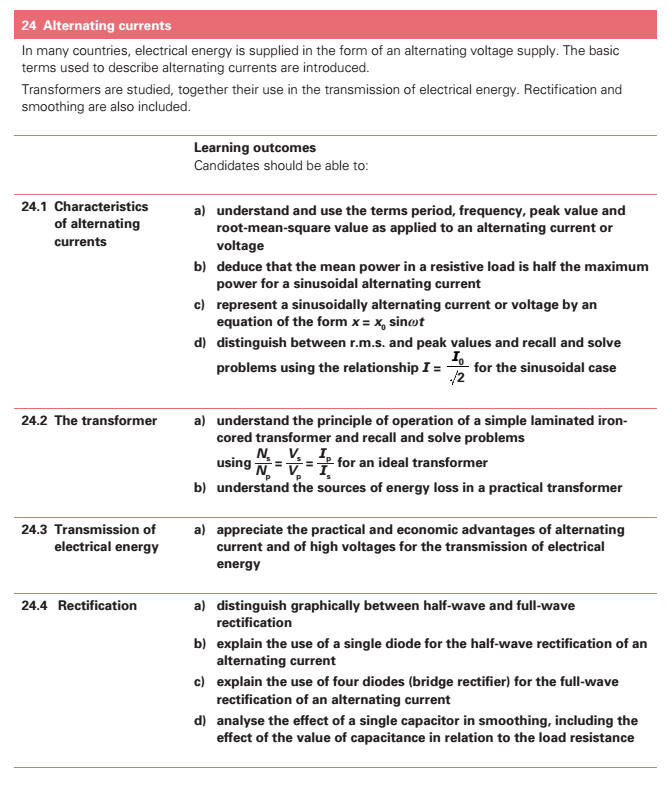18. Capacitance[] (a) show an understanding of the function of capacitors in simple circuits[]. (b) define capacitance[] and the farad. (c) recall and solve problems using C = Q/V. (d) derive, using the formula C = Q/V, conservation of charge and the addition of p.ds, formulae for capacitors in series and in parallel. (e) solve problems using formulae for capacitors in series and in parallel. * (f) deduce from the area under a potential-charge graph, the equation W = ½QV and hence W = ½CV2 . 22. Magnetic Fields[] (a) show an understanding that a magnetic field is an example of a field of force produced either by current-carrying conductors or by permanent magnets. (b) represent a magnetic field by field lines. 22 Electromagnetism (a) show an appreciation that a force might act on a current-carrying conductor placed in a magnetic field. (b) recall and solve problems using the equation F = BIl sinθ, with directions as interpreted by Flemings left-hand rule. (c) define magnetic flux density[] and the tesla. (d) show an understanding of how the force on a current-carrying conductor can be used to measure the flux density[] of a magnetic field using a current balance. (e) predict the direction of the force on a charge moving in a magnetic field. (f) recall and solve problems using F = BQv sinθ. (g) sketch flux patterns due to a long straight wire, a flat circular coil and a long solenoid. (h) show an understanding that the field due to a solenoid may be influenced by the presence of a ferrous core. (i) explain the forces between current-carrying conductors and predict the direction of the forces. (j) describe and compare the forces on mass, charge and current in gravitational, electric and magnetic fields[], as appropriate. 23. Electromagnetic Induction (a) define magnetic flux[] and the weber. (b) recall and solve problems using Φ = BA. (c) define magnetic flux[] linkage. * (d) infer from appropriate experiments on electromagnetic induction: (i) that a changing magnetic flux[] can induce an e.m.f. in a circuit, (ii) that the direction of the induced e.m.f. opposes the change producing it, (iii) the factors affecting the magnitude of the induced e.m.f. (e) recall and solve problems using Faradays law of electromagnetic induction and Lenzs law. (f) explain simple applications of electromagnetic induction. 24. Alternating Currents (a) show an understanding of and use the terms period[], frequency, peak value and root-mean-square value as applied to an alternating current or voltage. * (b) deduce that the mean power[] in a resistive load is half the maximum power[] for a sinusoidal alternating current. * (c) represent a sinusoidally alternating current or voltage by an equation of the form x = xosinωt . (d) distinguish between r.m.s. and peak values and recall and solve problems using the relationship  / 2 rms o I = I for the sinusoidal case. (e) show an understanding of the principle of operation of a simple iron-cored transformer and recall and solve problems using Ns/Np = Vs/Vp = Ip /Is for an ideal transformer[]. (f) show an appreciation of the scientific and economic advantages of alternating current and of high voltages for the transmission of electrical energy[]. * (g) distinguish graphically between half-wave and full-wave rectification. (h) explain the use of a single diode for the half-wave rectification[] of an alternating current. (i) explain the use of four diodes (bridge rectifier) for the full-wave rectification[] of an alternating current. * (j) analyse the effect of a single capacitor[] in smoothing, including the effect of the value of capacitance[] in relation to the load resistance[]